Dodging preserves the vertical position of an geom while adjusting the horizontal position. position_dodge() requires the grouping variable to be be specified in the global or geom_* layer. Unlike position_dodge(), position_dodge2() works without a grouping variable in a layer. position_dodge2() works with bars and rectangles, but is particulary useful for arranging box plots, which can have variable widths.

## Usage

position_dodge(width = NULL, preserve = c("total", "single"))

position_dodge2(
width = NULL,
preserve = c("total", "single"),
reverse = FALSE
)

## Arguments

width

Dodging width, when different to the width of the individual elements. This is useful when you want to align narrow geoms with wider geoms. See the examples.

preserve

Should dodging preserve the total width of all elements at a position, or the width of a single element?

Padding between elements at the same position. Elements are shrunk by this proportion to allow space between them. Defaults to 0.1.

reverse

If TRUE, will reverse the default stacking order. This is useful if you're rotating both the plot and legend.

Other position adjustments: position_identity(), position_jitterdodge(), position_jitter(), position_nudge(), position_stack()

## Examples

ggplot(mtcars, aes(factor(cyl), fill = factor(vs))) +
geom_bar(position = "dodge2")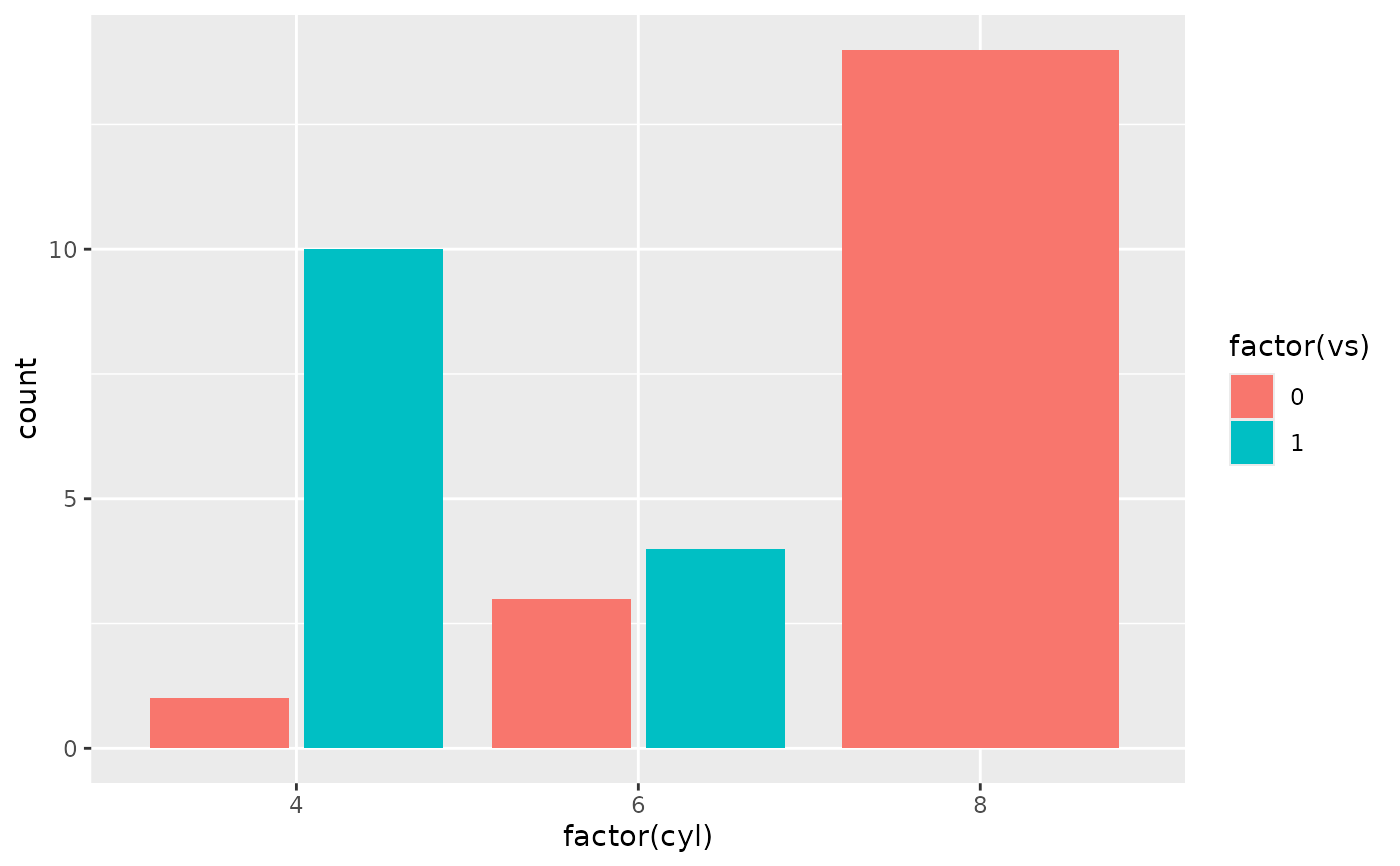# By default, dodging with position_dodge2() preserves the total width of
# the elements. You can choose to preserve the width of each element with:
ggplot(mtcars, aes(factor(cyl), fill = factor(vs))) +
geom_bar(position = position_dodge2(preserve = "single"))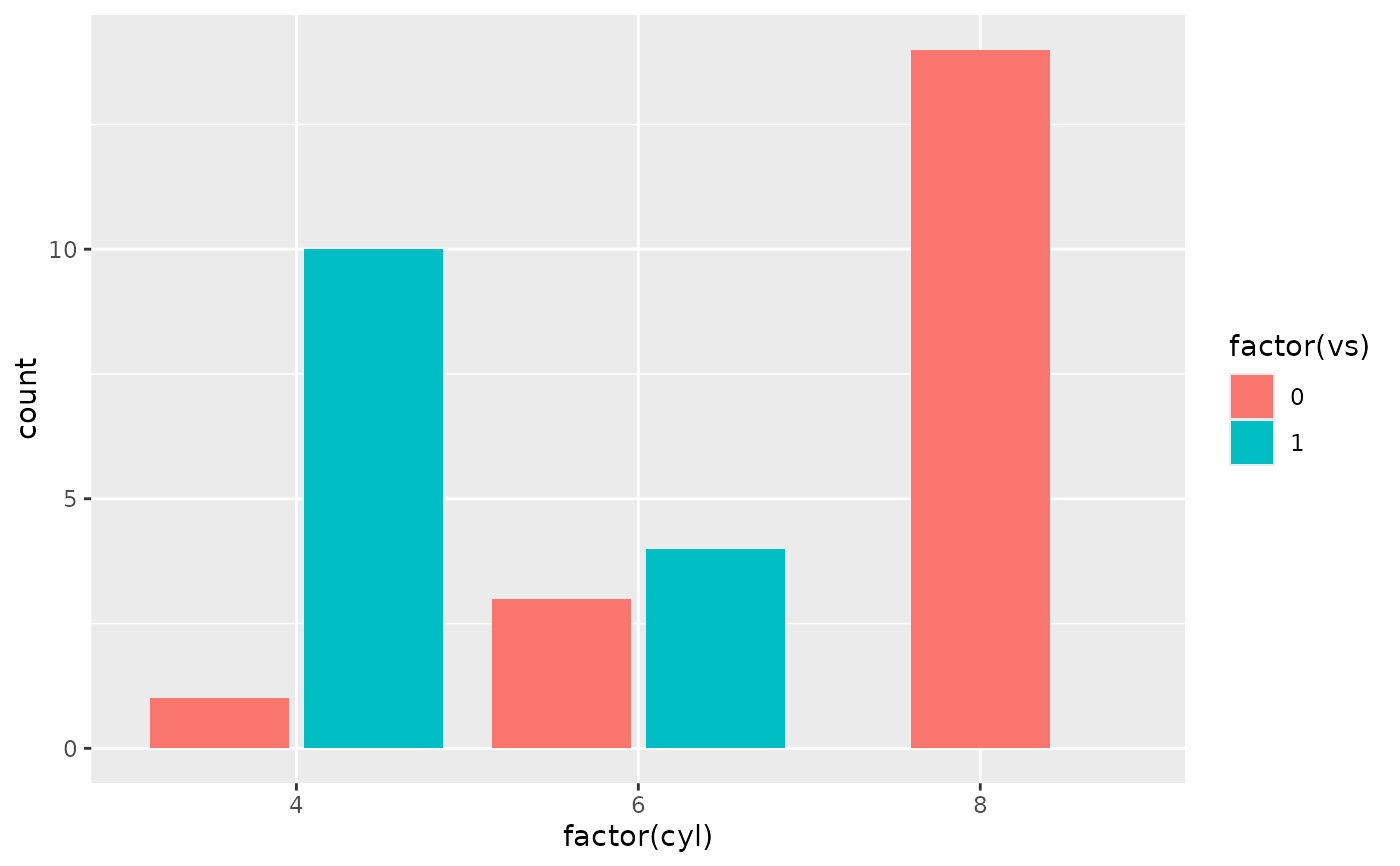# \donttest{
ggplot(diamonds, aes(price, fill = cut)) +
geom_histogram(position="dodge2")
#> stat_bin() using bins = 30. Pick better value with binwidth.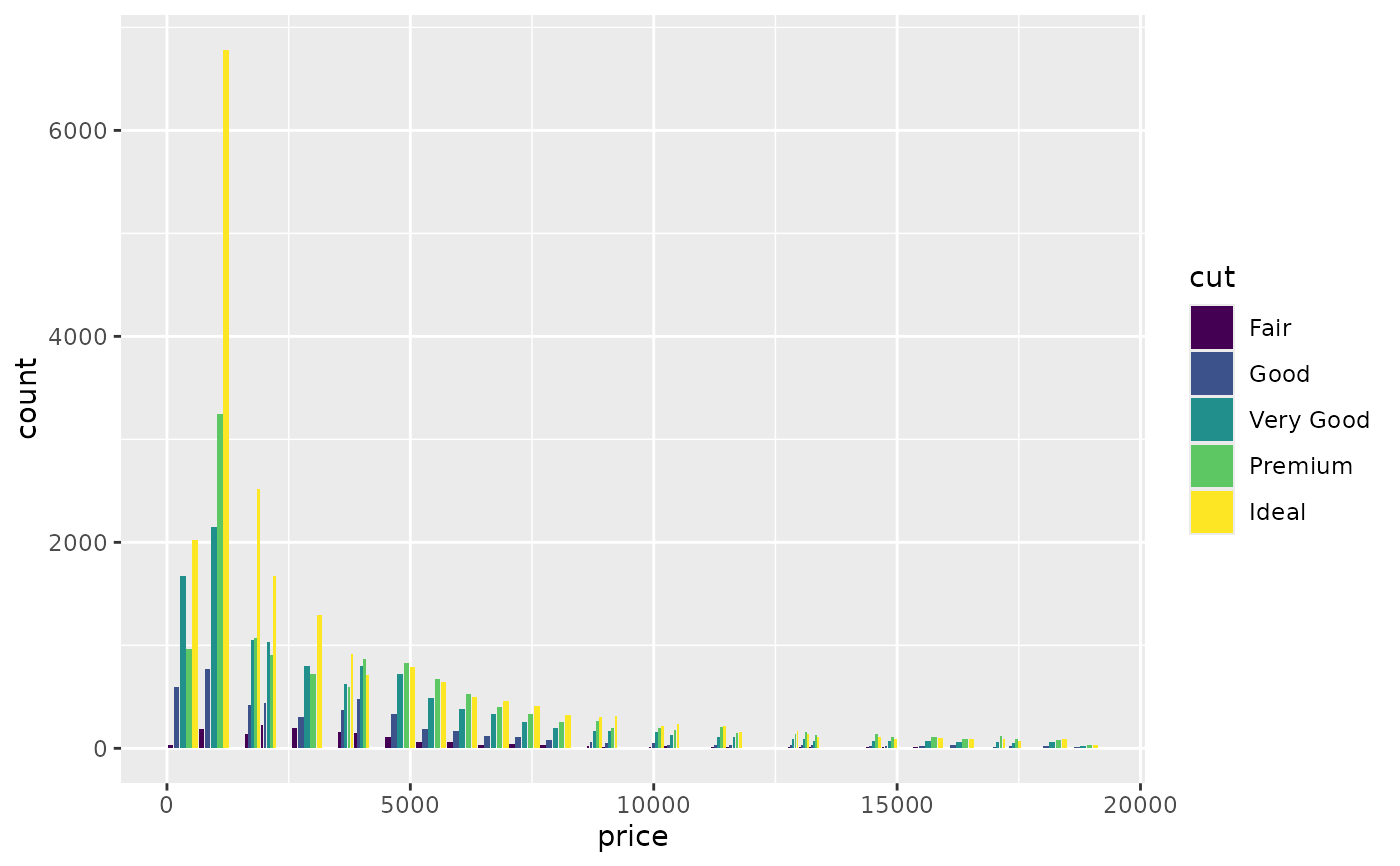# see ?geom_bar for more examples

# In this case a frequency polygon is probably a better choice
ggplot(diamonds, aes(price, colour = cut)) +
geom_freqpoly()
#> stat_bin() using bins = 30. Pick better value with binwidth.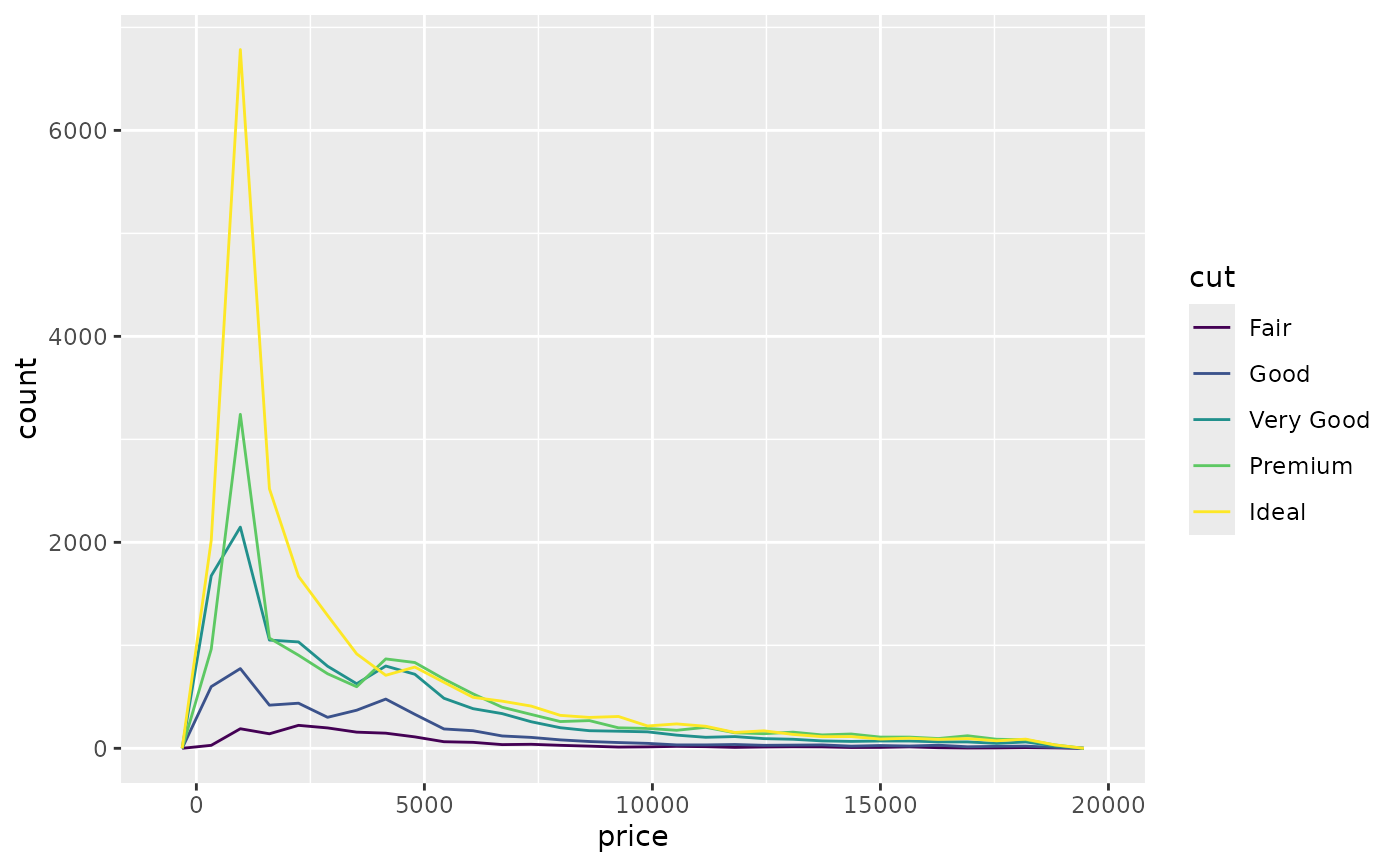# }

# Dodging with various widths -------------------------------------
# To dodge items with different widths, you need to be explicit
df <- data.frame(
x = c("a","a","b","b"),
y = 2:5,
g = rep(1:2, 2)
)
p <- ggplot(df, aes(x, y, group = g)) +
geom_col(position = "dodge", fill = "grey50", colour = "black")
p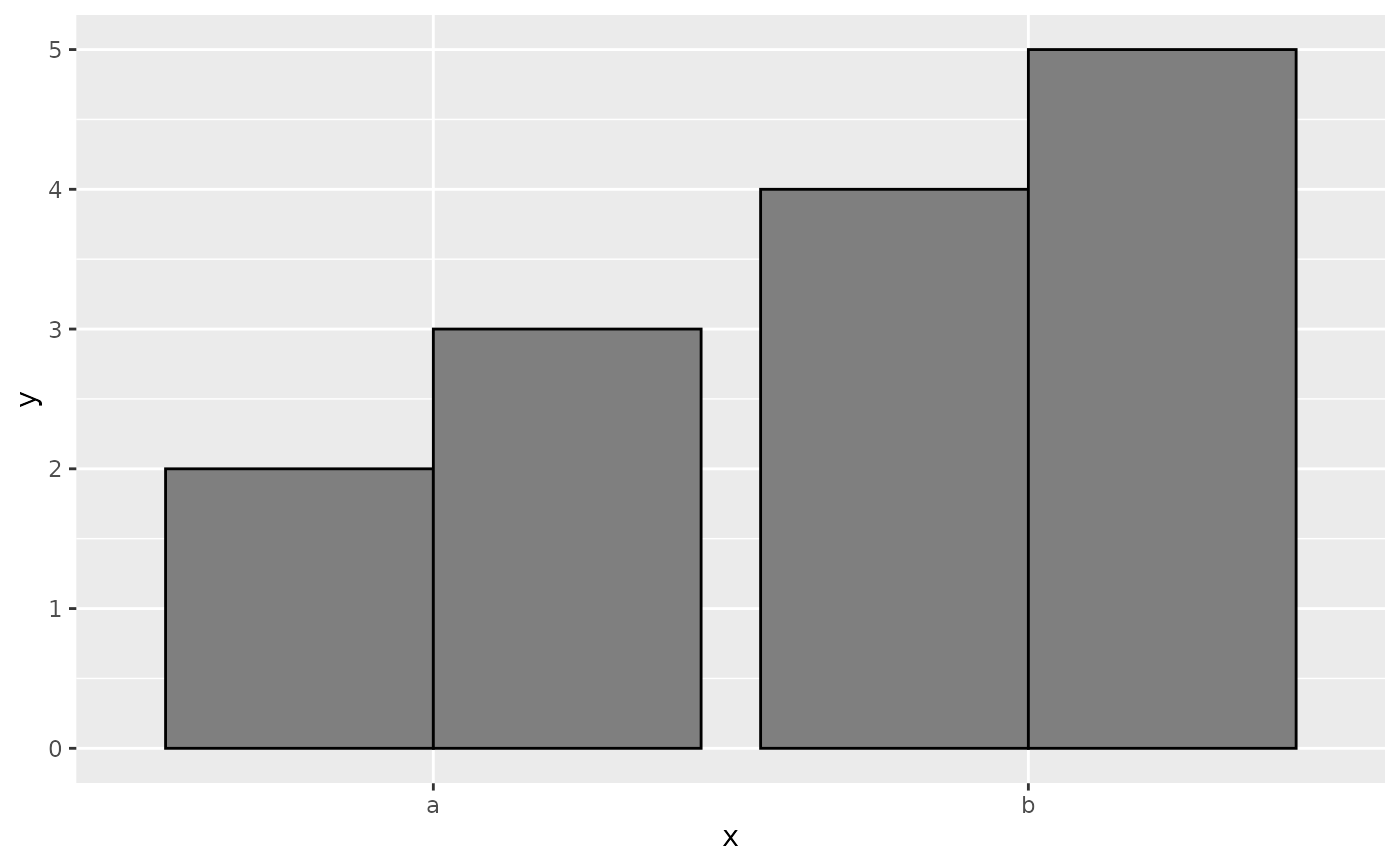# A line range has no width:
p + geom_linerange(aes(ymin = y - 1, ymax = y + 1), position = "dodge")
#> Warning: Width not defined. Set with position_dodge(width = ?)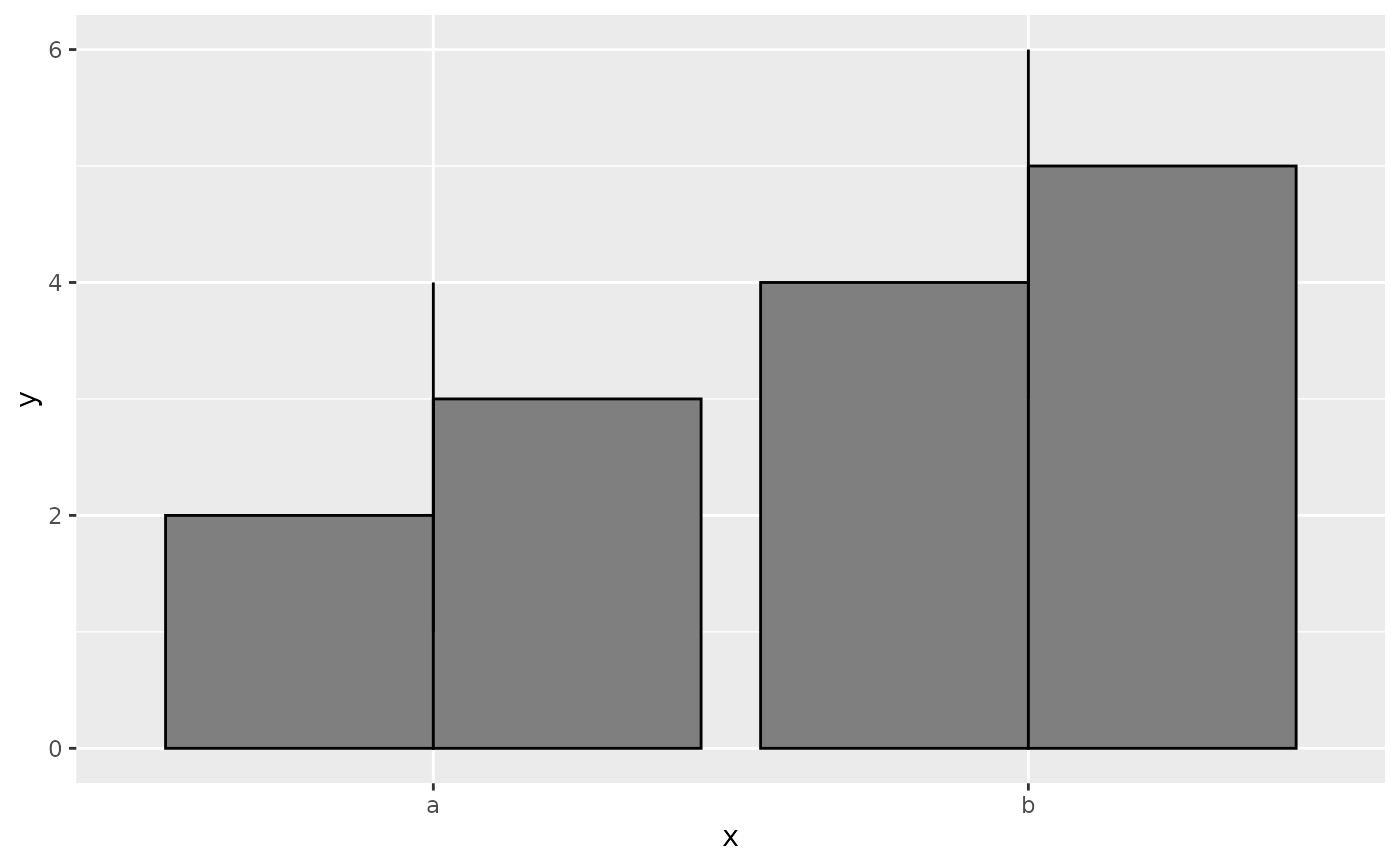# So you must explicitly specify the width
p + geom_linerange(
aes(ymin = y - 1, ymax = y + 1),
position = position_dodge(width = 0.9)
)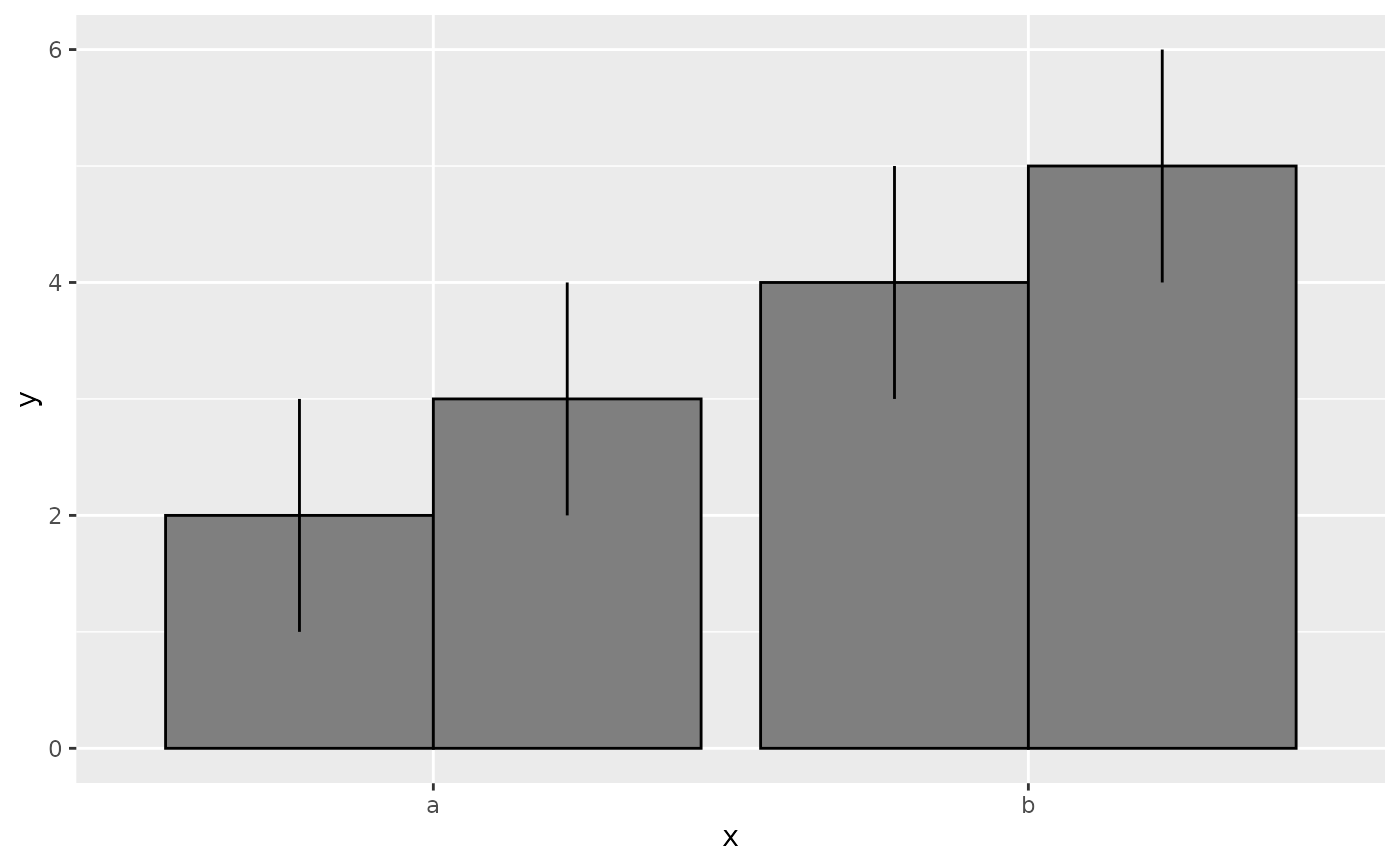# The same principle applies to error bars, which are usually
# narrower than the bars
p + geom_errorbar(
aes(ymin = y - 1, ymax = y + 1),
width = 0.2,
position = "dodge"
)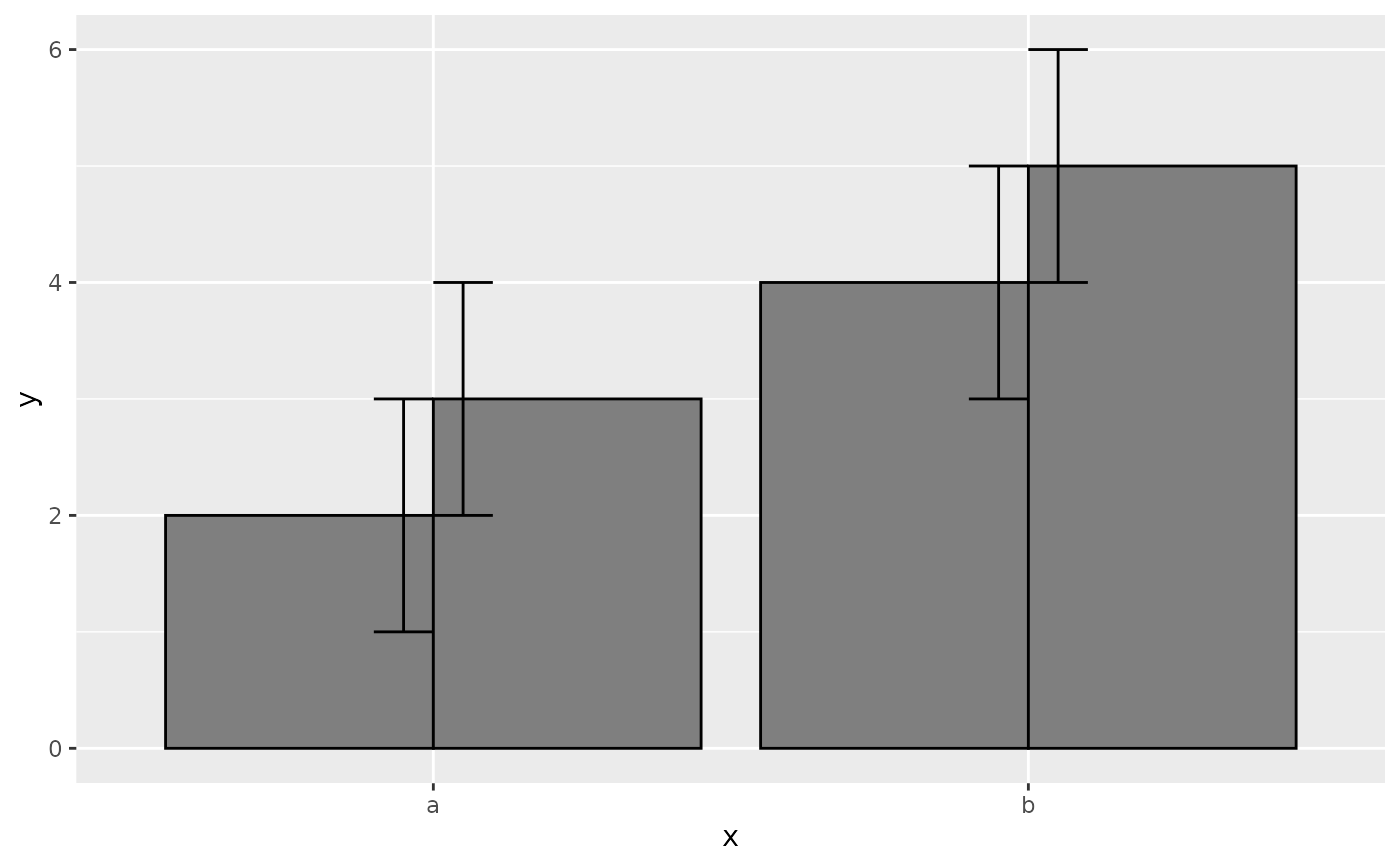p + geom_errorbar(
aes(ymin = y - 1, ymax = y + 1),
width = 0.2,
position = position_dodge(width = 0.9)
)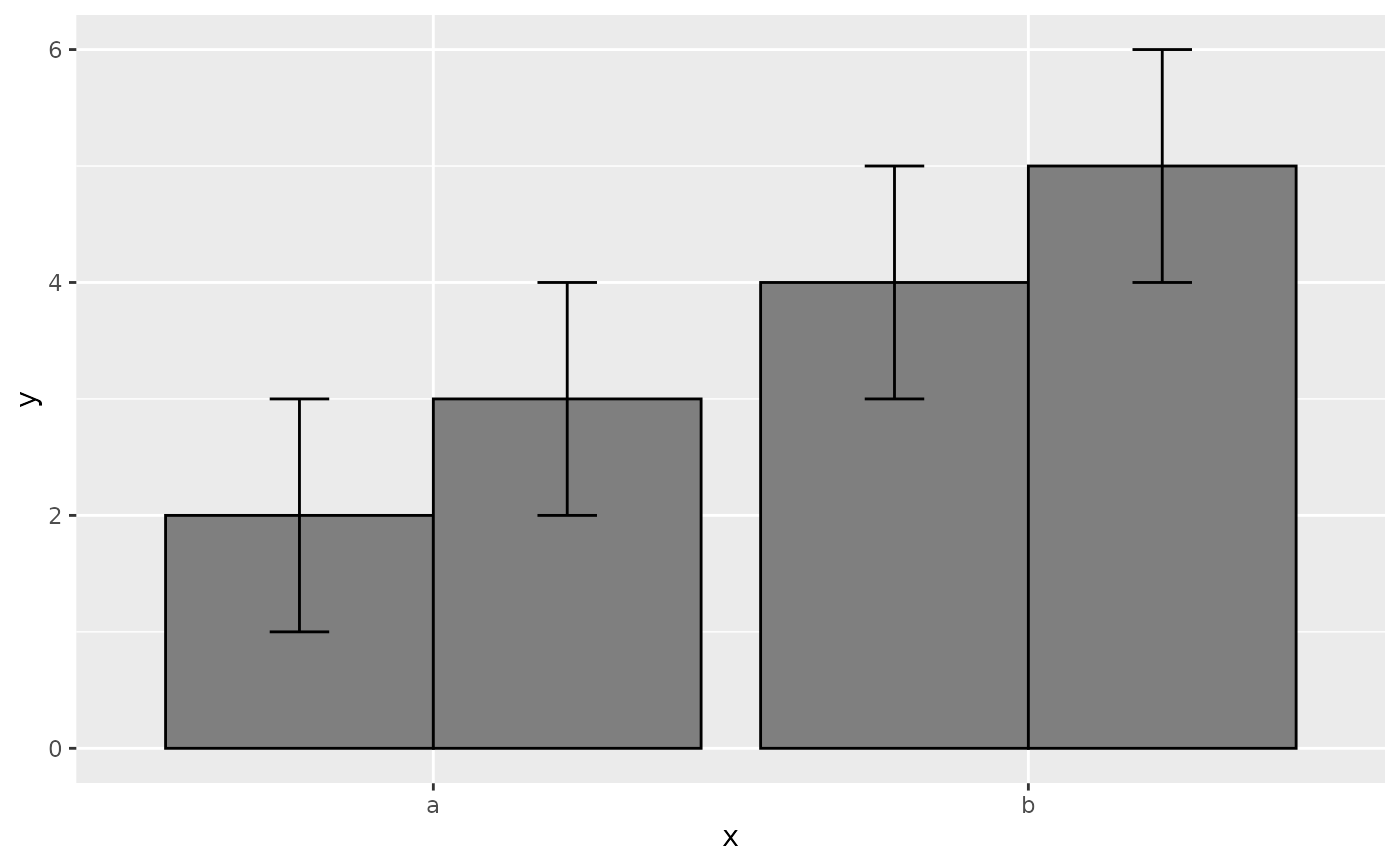# Box plots use position_dodge2 by default, and bars can use it too
ggplot(mpg, aes(factor(year), displ)) +
geom_boxplot(aes(colour = hwy < 30))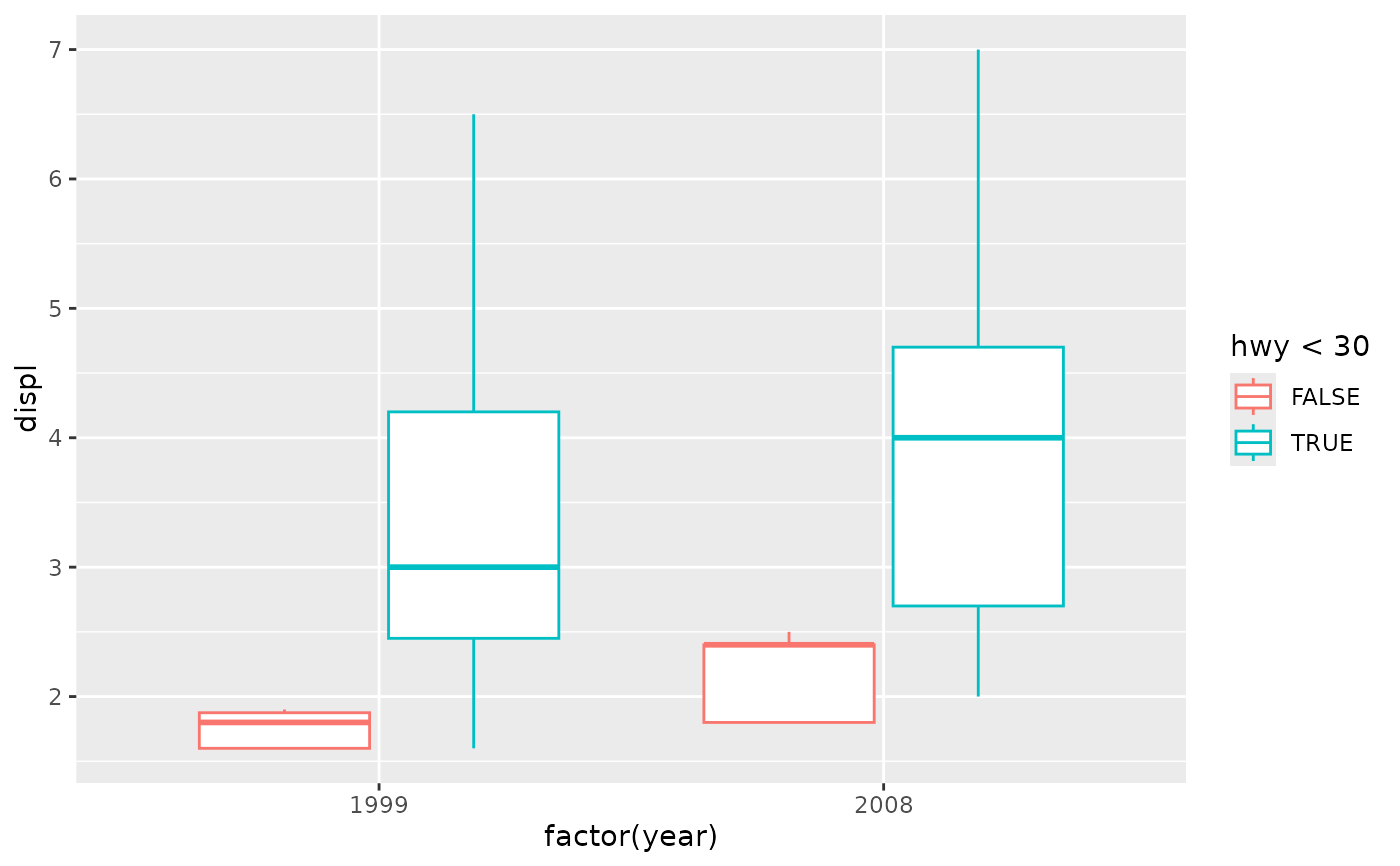ggplot(mpg, aes(factor(year), displ)) +
geom_boxplot(aes(colour = hwy < 30), varwidth = TRUE)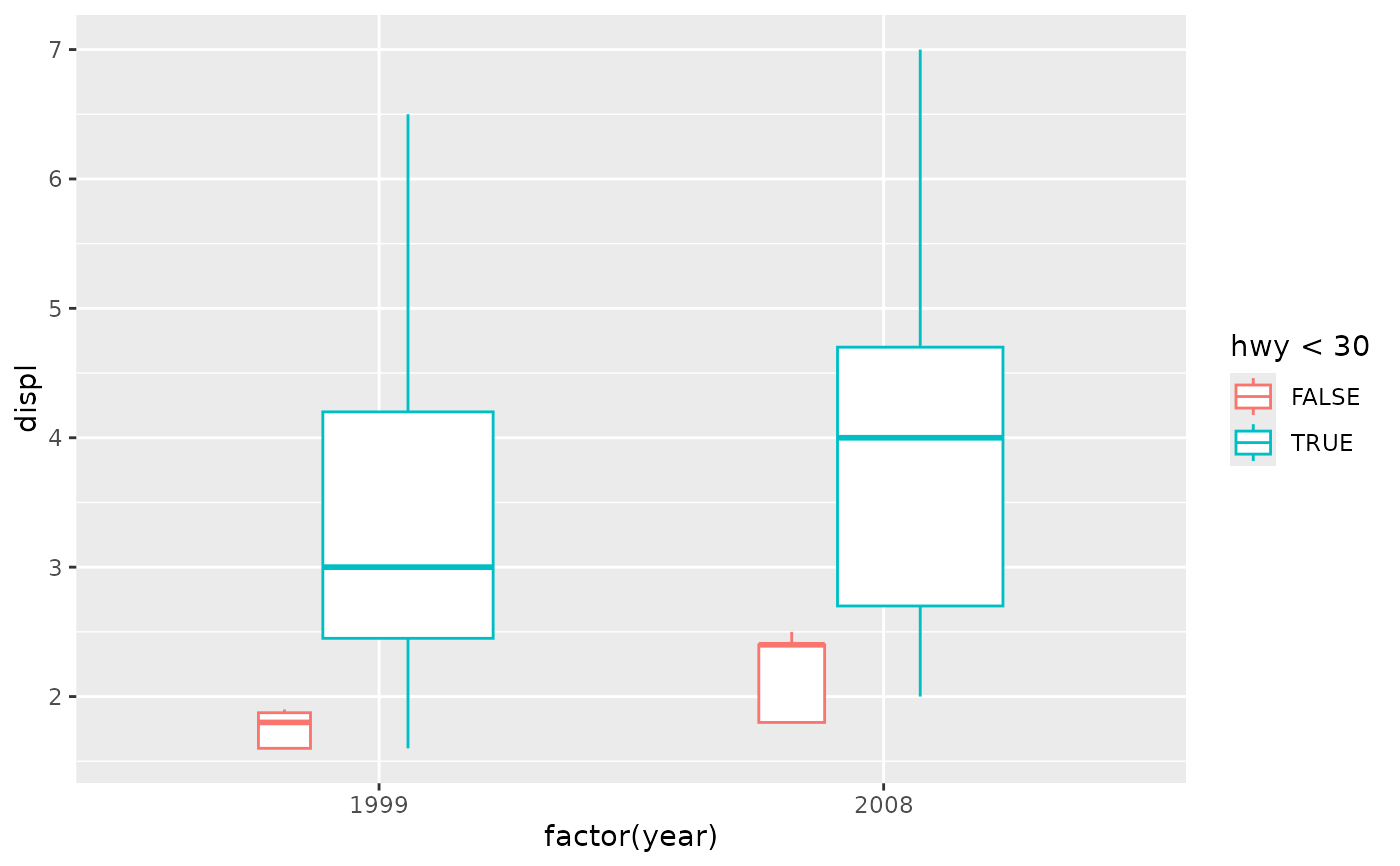ggplot(mtcars, aes(factor(cyl), fill = factor(vs))) +
geom_bar(position = position_dodge2(preserve = "single"))ggplot(mtcars, aes(factor(cyl), fill = factor(vs))) +
geom_bar(position = position_dodge2(preserve = "total"))